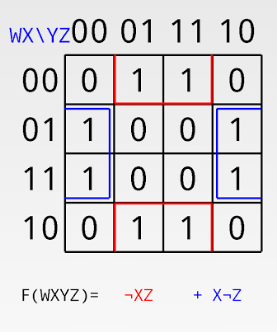Open In App

# GATE | GATE-CS-2007 | Question 85

Consider the following Boolean function of four variables:
f(w,x,y,z) = ∑(1,3,4,6,9,11,12,14)
The function is:
(A) independent of one variables.
(B) independent of two variables.
(C) independent of three variables.
(D) dependent on all the variables.

Explanation: